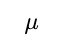A particle moves in the X-Y plane under the influence of a force such that its linear momentum is p(t ) = A[i cos(kt ) - j sin(kt )] , where A and k are constants. The angle between the force and the momentum is?

Anonymous User Physics Laws of Motion and Friction 17 Jul, 2020 75 views

A block is moving on an inclined plane making an angle 45° with the horizontal and the coefficient of friction is. The force required to just push it up the inclined plane is 3 times the force required to just prevent it from sliding down. If we define N = 10, then N is

Anonymous User Physics Laws of Motion and Friction 15 Jul, 2020 85 views